# 超好玩！这些功能用Python也能实现！五个场景教你用代码处理图像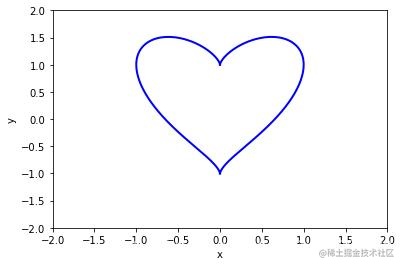# 前言

Python像是一个百宝箱，涵盖了很多宝贝，几乎什么方面都有所涉及。所以非常适合对Python零基础的小伙伴去初步探索、学习，你会发现这门语言并没有看上去那么难，并且也会领悟到他的精妙绝伦。## 1.词云图

python也有专门制作词云的库**-wordcloud**，能自定义颜色和形状。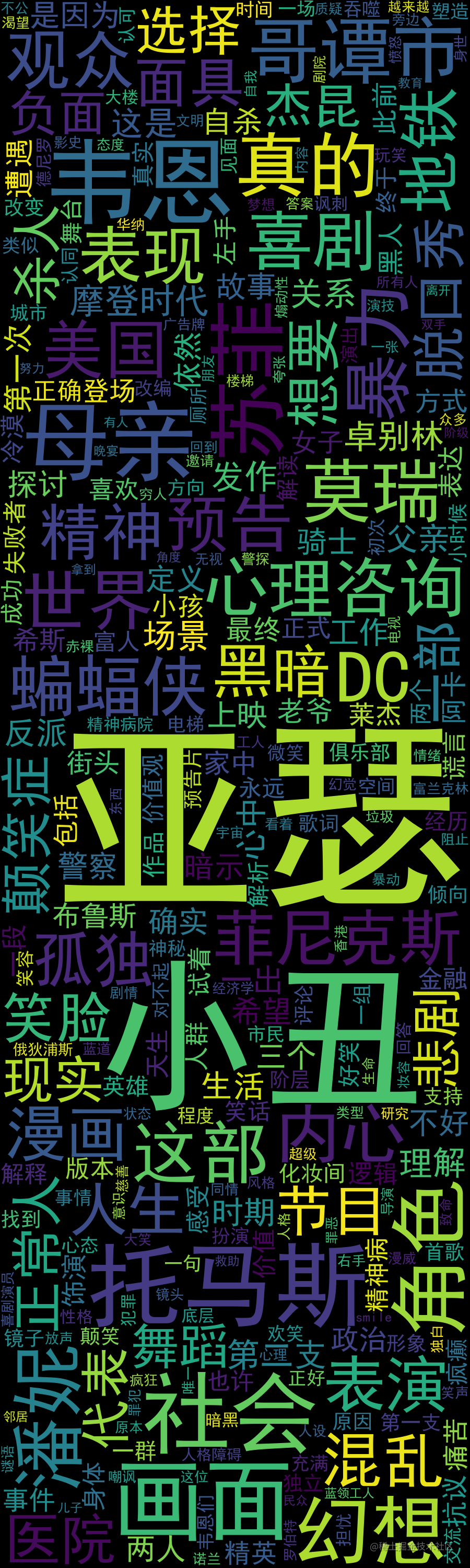``````def wordCloudImage(wordlist,width,height,bgcolor,savepath):
# 可以打开你喜欢的词云展现背景图
# 定义词云的一些属性
wc = WordCloud(
width=width,  # 图幅宽度 900
height=height,  # 图幅高度 3000
background_color=bgcolor,  # 背景图分割颜色为白色 "black"
max_words=300,  # 显示最大词数
font_path='./fonts/simhei.ttf',  # 显示中文
collocations=False,
# min_font_size=5,  # 最小尺寸
# max_font_size=100,  # 最大尺寸
)

# wordfile是分词后的词汇列表
x = wc.generate(wordlist)
# 生成词云图片
image = x.to_image()
# 展示词云图片
image.show()
# savepath是图片保存地址，保存词云图片
wc.to_file(savepath)

## 2. 生成手绘图片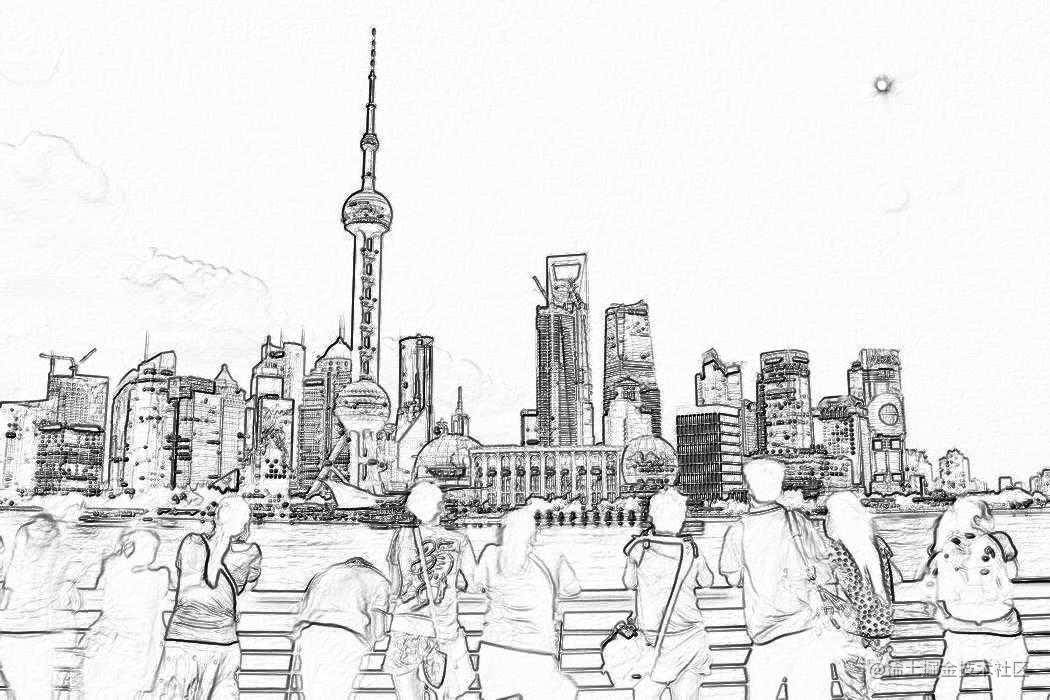``````# -*- coding: UTF-8 -*-
from PIL import Image
import numpy as np

# 原始图片路径
original_image_path = "E:\\图片\\陆家嘴.jpg"
# 要生成的手绘图片路径，可自定义
handdrawn_image_path = "E:\\图片\\陆家嘴-手绘.jpg"

# 加载原图，将图像转化为数组数据
a=np.asarray(Image.open(original_image_path).convert('L')).astype('float')
depth=10.

#取图像灰度的梯度值

#取横纵图像梯度值
uni_z=1./A

#光源的俯视角度转化为弧度值
vec_el=np.pi/2.2

#光源的方位角度转化为弧度值
vec_az=np.pi/4.

#光源对x轴的影响
dx=np.cos(vec_el)*np.cos(vec_az)
dy=np.cos(vec_el)*np.sin(vec_az)
dz=np.sin(vec_el)

#光源归一化，把梯度转化为灰度
b=255*(dx*uni_x+dy*uni_y+dz*uni_z)

#避免数据越界，将生成的灰度值裁剪至0-255内
b=b.clip(0,255)

#图像重构
im=Image.fromarray(b.astype('uint8'))

print('完成')
im.save(handdrawn_image_path)

## 3、生成艺术二维码``````myqr 网址链接

``````myqr https://zhuanlan.zhihu.com/pydatalysis

``````myqr https://zhuanlan.zhihu.com/pydatalysis -p d:\hmbb.jpg -c

## 4. 生成证件照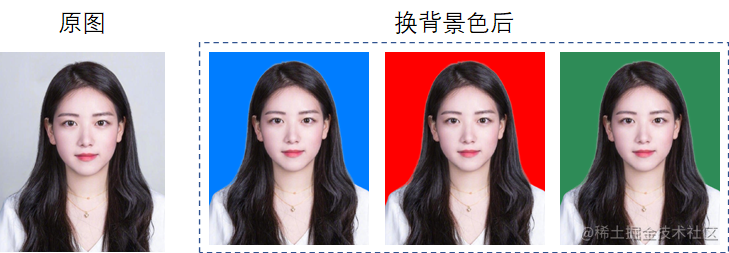``````# encoding=utf-8
from PIL import Image
from removebg import RemoveBg

# removebg涉及到api_key,需要到其官网申请
api_key = 'PysKLJueeoyK9NbJXXXXXXXXX'

def change_bgcolor(file_in, file_out, api_key, color):
'''
#必须为png格式
'''
p, s = file_in.split(".")
rmbg = RemoveBg(api_key, 'error.log')
rmbg.remove_background_from_img_file(file_in)
file_no_bg = "{}.{}_no_bg.{}".format(p, s, s)
no_bg_image = Image.open(file_no_bg)
x, y = no_bg_image.size
new_image = Image.new('RGBA', no_bg_image.size, color=color)
new_image.paste(no_bg_image, (0, 0, x, y), no_bg_image)
new_image.save(file_out)

# 修改照片尺寸
def change_size(file_in, file_out, width, height):
image = Image.open(file_in)
resized_image = image.resize((width, height), Image.ANTIALIAS)
resized_image.save(file_out)

if __name__ == "__main__":
file_in = 'E:\\girl.png'
file_out = 'E:\\girl_cutout.png'
# 尺寸可按需求自修改
# change_size(file_in, file_out, width, height)

# 换背景色
color = (0, 125, 255)
change_bgcolor(file_in, file_out, api_key, color)

## 5. 生成九宫格图片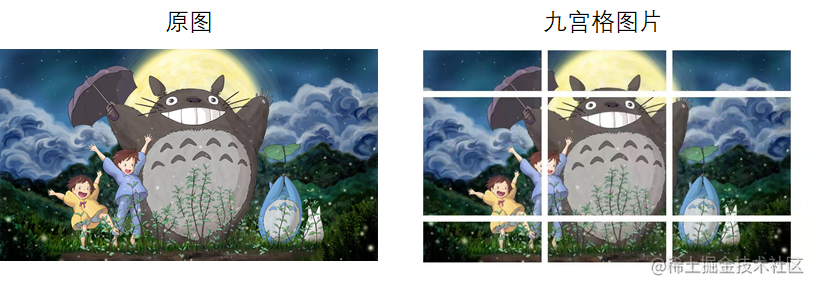代码：

``````# 朋友圈九宫格图片制作
# encoding=utf-8
from PIL import Image
import sys

# 先将input image 填充为正方形
def fill_image(image):
width, height = image.size
# 选取原图片长、宽中较大值作为新图片的九宫格半径
new_image_length = width if width > height else height
# 生产新图片【白底】
new_image = Image.new(image.mode, (new_image_length, new_image_length), color='white')
# 将原图粘贴在新图上，位置为居中
if width > height:
new_image.paste(image, (0, int((new_image_length - height) / 2)))
else:
new_image.paste(image, (int((new_image_length - width) / 2), 0))
return new_image

# 将图片切割成九宫格
def cut_image(image):
width, height = image.size
# 一行放3张图
item_width = int(width / 3)
box_list = []
for i in range(0, 3):
for j in range(0, 3):
box = (j * item_width, i * item_width, (j + 1) * item_width, (i + 1) * item_width)
box_list.append(box)
image_list = [image.crop(box) for box in box_list]
return image_list

# 保存图片
def save_images(image_list):
index = 1
for image in image_list:
image.save('e:\\图片\\'+str(index) + '.png', 'PNG')
index += 1

if __name__ == '__main__':
file_path = "e:\\图片\\龙猫.jpg"
image = Image.open(file_path)
# image.show()
image = fill_image(image)
image_list = cut_image(image)
print(len(image_list))
save_images(image_list)

#写在最后: